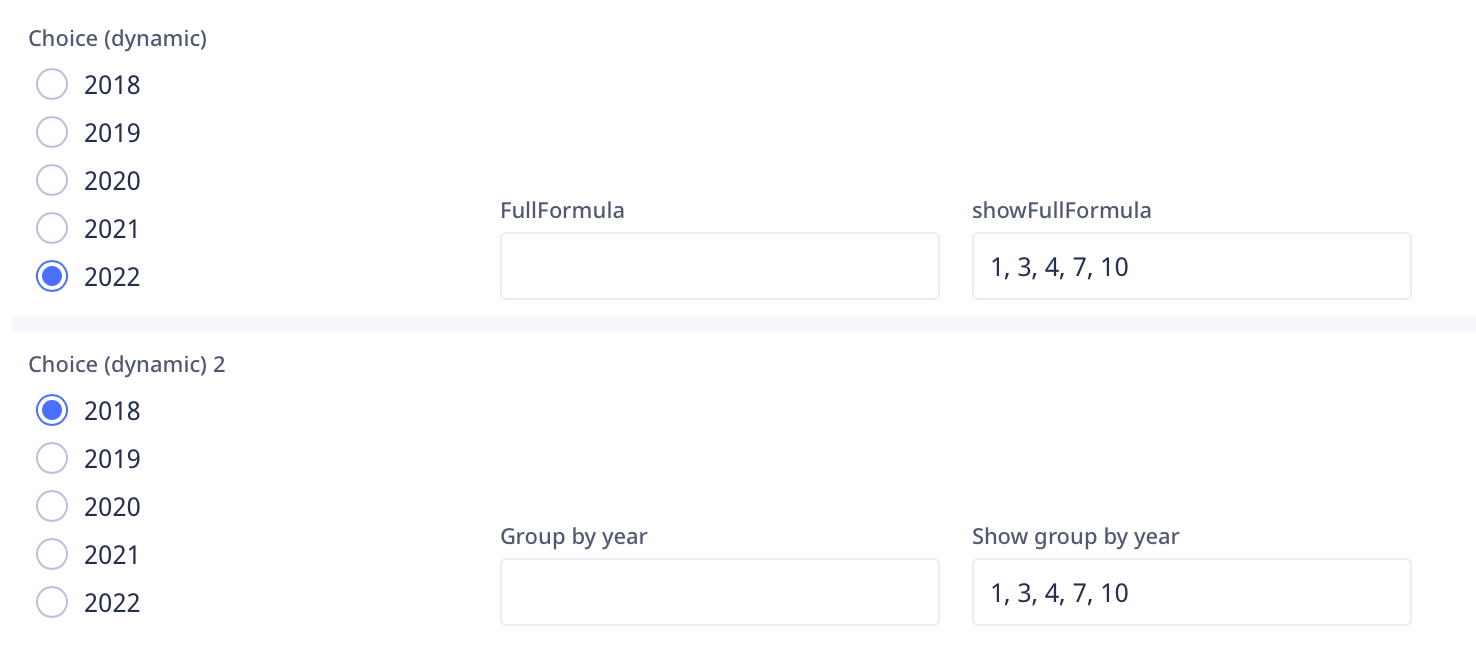2

# How to create a unique list of records

Let us say I have a table, Table1, with records that store years in a field called Number. Now I want to create a dynamic Choice field in Table2 that shows the unique years that are in Table1. Since we know dynamic fields need record level data to function we have to find a way to find all records but eliminate the records that are duplicate except for one.

Here is my one solution. I have attached a DB, that breaks down each step, and will post the full formula below.

``````#{
Replace: Table1 and Number to match your table and field names
Step 1: gather all the data from the table you need
}#;
let step1 := (select Table1);
#{
Step 2: filter the results in Step 1 to only the records with duplicate data.
step A: starting with the step2A variable that gets the data from the field that is duplicated.
step B: Then filters the step1 variable
}#;
let step2A := for loop1 in step1 do
loop1.Number
end;
let step2B := step1[(var N := Number;
count(step2A[= N])) > 1];
#{
Step 3: filter the step2B results to only show the first instance of each duplicated data.
step A: find the value that is duplicated and then get an unique array of the values
step B: using a for loop, find the first record in each set of records that have duplicate values
}#;
let step3A := unique(step2B.Number);
let step3B := for loop1 in step3A do
first(step2B[Number = loop1])
end;
#{
Step 4: Creating an array of records that should be removed from Step 1 since they are duplicates
}#;
let step4 := step2B[var t := this;
count(step3B[= t]) = 0];
#{
Step 5: take the array in Step 4 and remove it from the array in Step 1 and you are left with a list of records that have a unique value
}#;
step1[var t := this;
count(step4[= t]) = 0]
``````

The main issue I see is that you can't use the soft links that dynamic choice fields allow you to create, but if all you want is a list then this could work.

Also if you don't want the first instance of a duplicate set then I'm not sure what you would do, but I'm sure others will figure something out.

Look forward to seeing what others come up with.

## 4replies• UweG
• 10 mths ago
• Reported - view

Wow!!!

• Fred
• 10 mths ago
• Reported - view

I’ve wanted to do something like this for about a year now.

• Jacques_TUR
• 10 mths ago
• Reported - view

Thanks to Fred for inviting us to look into how to create a function equivalent to group in SQL. It's a good exercise and it can be really useful.
My solution looks like this. Does it match your project?

``````var all := (select Table1);
for i in unique(all.Number) do
first(all[Number = i])
end
``````

I do it with your database and I get the same result:• Fred
• 10 mths ago
• Reported - view

Well Jacques, your code makes a lot of sense and is much easier to use.

## Content aside

• 2 Likes
• 10 mths agoLast active
• 4Replies
• 402Views
• 3 Following